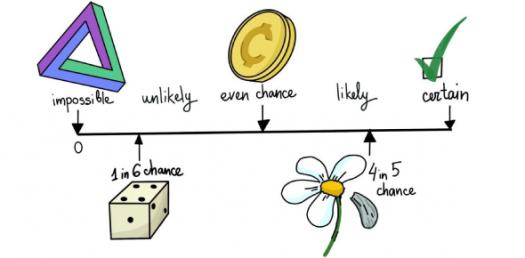Check How Much You Can Score In The Probability Test? Quiz!

18 Questions | Total Attempts: 255SettingsCheck how much you can score in the probability test? Do you have a hard time when it comes to solving questions on probabilities and is looking for a quiz to give you some much-needed practice? Worry not as this quiz is perfect for both beginners and people who fancy random math exercises. Do give it a shot!

• 1.
A bag contains 4 blue marbles, 6 red marbles, 10 yellow marbles and 6 green marbles. What is the probability of not drawing a red marble?
• A.

6/26

• B.

3/13

• C.

2/13

• D.

10/13

• 2.
Use the diagram below to determine if the following statement is true or false. The probability of someone liking neither horses nor cows is 4/25.
• A.

True

• B.

False

• 3.
Determine the probability of spinning a green on the following spinner:
• A.

1/8

• B.

1/6

• C.

1/4

• D.

2/8

• 4.
There are 20 marbles in a bag, 4 blue, 4 green, 4 red, 4 white and 4 black.  Remember that all fraction answers should be given in the simplest form. What is the probability of drawing a blue marble?
• A.

1/20

• B.

4/20

• C.

4/5

• D.

1/5

• 5.
There are 20 marbles in a bag, 4 blue, 4 green, 4 red, 4 white and 4 black.  Remember that all fraction answers should be given in the simplest form. What is the probability of drawing a black or white marble?
• A.

2/3

• B.

8/12

• C.

8/20

• D.

2/5

• 6.
If you have a standard deck of cards (52 cards) what is the probability of drawing the Queen of Hearts?
• A.

1/51

• B.

51/1

• C.

52/1

• D.

1/52

• 7.
If a month is picked at random, what is the probability that the month will start with M?
• A.

1/12

• B.

1/6

• C.

2/6

• D.

4/12

• 8.
A spinner that is numbered 1-7 is used for a game. What is the probability that a 5 will be spun?
• A.

1/6

• B.

1/5

• C.

1/7

• D.

5/7

• 9.
A card game has 25 red cards, 25 green cards, 25 yellow cards, 25 blue cards, and 8 wild cards. What is the probability that the first card dealt is a wild card?
• A.

8/100

• B.

4/25

• C.

2/27

• D.

4/27

• 10.
For his garden, Clay has a mixture of 12 white corn seeds, 24 yellow corn seeds, and 16 bi-color corn seeds. If he reaches for a seed without looking, what is the probability that Clay will  plant a bi-color corn seed first?
• A.

4/13

• B.

1/13

• C.

6/13

• D.

4/52

• 11.
Suppose that you have a bag containing 3 red jelly beans, 4 green jelly beans, 5 yellow jelly beans, and 1 black jelly bean.  If you pick one jelly bean from the bag at random, what is the probability that the bean will be yellow?
• 12.
Suppose that you have a bag containing 3 red jelly beans, 4 green jelly beans, 5 yellow jelly beans, and 1 black jelly bean. If you pick one jelly bean, what is the probability that it will be either red or green?
• 13.
In a survey of 100 students about their favorite pizza topping, 25 chose pineapple. According to these results, if a student is picked at random at the school, what is the chance that the student prefers pineapple as a pizza topping?
• A.

1 out of 4

• B.

1 out of 25

• 14.
Ben and Lily are going to roll a number cube one time to see who will start a game. Which of the following IS a fair way to decide who will go first?
• A.

If a 1, 4 or 6 is rolled, Ben will go first. If a 2,3 or 5 is rolled, Lily will go first.

• B.

If the number rolled is less than 3, Ben will go first. If the number rolled is greater than 3, Lily will go first.

• 15.
The probability of rolling a 6 on a regular number cube is 1/6. If Jacob tosses a number cube 48 times, how many times would he expect to roll a 6?
• A.

8

• B.

6

• 16.
A standard number cube with the numbers 1 through 6 is rolled. Find the probability of rolling a number LESS THAN 2.
• A.

1/6

• B.

1/3

Related TopicsBack to top